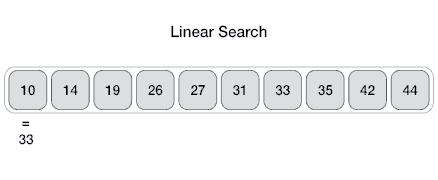Need help with Dart?
Click the “chat” button below for chat support from the developer who created it, or find similar developers for support.#### About the developerTheAlgorithms279 Stars117 ForksMIT License538 Commits7 Opened issues!
?

#### Need anything else?# The Algorithms - Dart

### All algorithms implemented in Dart (for education)

These implementations are for learning purposes. They may be less efficient than the implementations in the Dart standard library.

## List of Algorithms

See our directory for full list of all algorithms. A few of the algorithms (the most common ones) are explained here.

## Search Algorithms

### LinearFrom Wikipedia: linear search or sequential search is a method for finding a target value within a list. It sequentially checks each element of the list for the target value until a match is found or until all the elements have been searched. Linear search runs in at the worst linear time and makes at most n comparisons, where n is the length of the list.

Properties * Worst case performance O(n) * Best case performance O(1) * Average case performance O(n) * Worst case space complexity O(1) iterative

### BinaryFrom Wikipedia: Binary search, also known as half-interval search or logarithmic search, is a search algorithm that finds the position of a target value within a sorted array. It compares the target value to the middle element of the array; if they are unequal, the half in which the target cannot lie is eliminated and the search continues on the remaining half until it is successful.

Properties * Worst case performance O(log n) * Best case performance O(1) * Average case performance O(log n) * Worst case space complexity O(1)

## Sort Algorithms

### BubbleFrom Wikipedia: Bubble sort, sometimes referred to as sinking sort, is a simple sorting algorithm that repeatedly steps through the list to be sorted, compares each pair of adjacent items and swaps them if they are in the wrong order. The pass through the list is repeated until no swaps are needed, which indicates that the list is sorted.

Properties * Worst case performance O(n^2) * Best case performance O(n) * Average case performance O(n^2)

### InsertionFrom Wikipedia: Insertion sort is a simple sorting algorithm that builds the final sorted array (or list) one item at a time. It is much less efficient on large lists than more advanced algorithms such as quicksort, heapsort, or merge sort.

Properties * Worst case performance O(n^2) * Best case performance O(n) * Average case performance O(n^2)

### QuickFrom Wikipedia: Quicksort (sometimes called partition-exchange sort) is an efficient sorting algorithm, serving as a systematic method for placing the elements of an array in order.

Properties * Worst case performance O(n^2) * Best case performance O(n log n) or O(n) with three-way partition * Average case performance O(n^2)

### SelectionFrom Wikipedia: The algorithm divides the input list into two parts: the sublist of items already sorted, which is built up from left to right at the front (left) of the list, and the sublist of items remaining to be sorted that occupy the rest of the list. Initially, the sorted sublist is empty and the unsorted sublist is the entire input list. The algorithm proceeds by finding the smallest (or largest, depending on sorting order) element in the unsorted sublist, exchanging (swapping) it with the leftmost unsorted element (putting it in sorted order), and moving the sublist boundaries one element to the right.

Properties * Worst case performance O(n^2) * Best case performance O(n^2) * Average case performance O(n^2)

### MergeFrom Wikipedia: Merge sort (also commonly spelled mergesort) is a divide and conquer algorithm that was invented by John von Neumann in 1945. The algorithm dirst divides the list into the smallest unit (1 element), then compares each element with the adjacent list to sort and merge the two adjacent lists. Finally all the elements are sorted and merged. It is an efficient, general-purpose, comparison-based sorting algorithm.

Properties * Worst case performance O(n log n) * Best case performance O(n log n) * Average case performance O(n log n)

### ShellFrom Wikipedia: Shellsort is a generalization of insertion sort that allows the exchange of items that are far apart. The idea is to arrange the list of elements so that, starting anywhere, considering every nth element gives a sorted list. Such a list is said to be h-sorted. Equivalently, it can be thought of as h interleaved lists, each individually sorted.

Properties * Worst case performance O(nlog2 2n) * Best case performance O(n log n) * Average case performance depends on gap sequence

### Time-Complexity Graphs

Comparing the complexity of sorting algorithms (Bubble Sort, Insertion Sort, Selection Sort)

Complexity Graphs

## Community Channel

We're on Gitter! Please join us.

## Contribution

Please read our CONTRIBUTING.md.

## License

MIT

We use cookies. If you continue to browse the site, you agree to the use of cookies. For more information on our use of cookies please see our Privacy Policy.